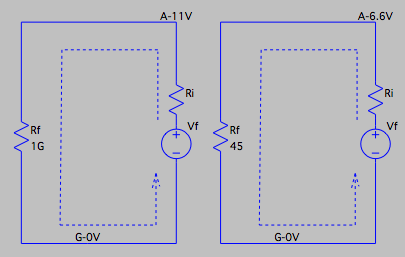Check the Filament Voltage by Ohm's Law Applied to a Circuit in Series

 DC circuit of the tube filament, for analysis. Look at the two circuits on the left, which are the circuits for heating the filament of the tube. The two circuits are almost the same. Vf represents the voltage source from the PSU, with an unknown output voltage. The resistor Ri is the unknown inner resistor of the source. Every voltage source has an inner resistance. But its value is unknown yet. Rf is the resistor representing the resistance of the tube filament. The difference in the two circuits is the value of the Rf. On the left, Rf=1G, which is very large, representing the filament is not connected, or broken. On the right, Rf=45, which is in the normal range of a tube filament. We also measured the voltage at the point A. With Rf=1G, Va=11V, and with Rf=45, Va=6.6V. What is the use of these voltage values at A? First of all, the voltage at A must be around 6.6V. If it is around 11V, we are sure the filament of the tube is not connected. The most common reason is the bad connection of the tube PCB to the main PCB. Of cause, another reason might be that the filament is broken, but it happened not very often. When the voltage at A is 11V, we know the output voltage for the filament from the PSU is 11V. Why? Let us apply the Ohm's law to the circuit shown on the left with Rf=1G. First we calculate the DC current through Ri and Rf using Ohm's law. I = Vf / (Ri + Rf) Then we calculate the voltage across the resistor Ri, also by Ohm's law, Vri = Ri * I Now we can get the voltage at A as Va = Vf - Vri = Vf - Ri * Vf / (Ri + Rf) = Vf * (1 - Ri/(Ri+Rf)) = Vf * Rf / (Ri + Rf) We do not know the value of Ri yet, but we can be very certain that compared to 1G, it is nothing. So Ri+Rf=Rf, and Rf/Rf=1. Then we get Va=Vf in this case. We know the output voltage for the filament from the PSU is 11V. When the voltage at A is 6.6V, we know the inner resistance of the voltage source is 30 Ω. Why? Since we know the voltage across Rf is 6.6V, we can get the current through it I = Va / Rf = 6.6 / 45 = 150 mA This current is the same as the one through Ri, so the Ri should be Ri = (Vf - Va) / I = (11 - 6.6) / 0.15 = 30 Ω